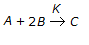# Chemical Engineering - Chemical Reaction Engineering

### Exercise :: Chemical Reaction Engineering - Section 1

6.

What is the order of a chemical reaction,, if the rate of formation of 'C', increases by a factor of 2.82 on doubling the concentration of 'A' and increases by a factor of 9 on trebling the concentration of 'B'?

 A. 7/2 B. 7/4 C. 5/2 D. 5/4

Explanation:

No answer description available for this question. Let us discuss.

7.

For high conversion in a highly exothermic solid catalysed reaction, use a __________ bed reactor.

 A. fixed B. fluidised bed reactor followed by a fixed C. fixed bed reactor followed by a fluidised D. fluidised

Explanation:

No answer description available for this question. Let us discuss.

8.

For every 10°C rise in temperature, the rate of chemical reaction doubles. When the temperature is increased from 30 to 70°C, the rate of reaction increases __________ times.

 A. 8 B. 12 C. 16 D. 32

Explanation:

No answer description available for this question. Let us discuss.

9.

The single parameter model proposed for describing non-ideal flow is the __________ model.

 A. tank in series B. dispersion C. both (a) & (b) D. neither (a) nor (b).

Explanation:

No answer description available for this question. Let us discuss.

10.

A first order reaction requires two equal sized CSTR. The conversion is

 A. less when they are connected in series. B. more when they are connected in series. C. more when they are connected in parallel. D. same whether they are connected in series or in parallel.